Courses
Courses for Kids
Free study material
Offline Centres
More

# Easy Tricks to Solve Multiplication Sums with StoriesLast updated date: 27th Nov 2023
Total views: 181.8k
Views today: 3.81k## Multiplication Sums - Story Problems Maths, Grade-4

Do you know an easy trick to solve multiplication story sums? Let us assume there are 5 students in Class 4 in each section- A, B, C, and D. If you have to find the total number of students in all four sections, you can just do 5 times 4, i.e., 5 * 4 = 20 students in total.

However, another way to find the total number of students is, just add 5 + 5 + 5 + 5, you get 20 again. Awesome isn’t it? Well, here, we notice that multiply sums can be done by performing the addition of numbers. Multiplication is one of the interesting concepts of maths. Here, you will learn interesting tricks on solving multiplication problems. Let’s go through Saumya’s story and understand how multiplication works.

### Story of Water Supply in ABC Society

During summers, water supply is a major problem in ABC society. The water supply starts at 8: 00 a.m. and ends at 9: 00 p.m., which means 13 hrs supply, the same is continued each day. Now, if I say how many hours did the water supply was there in a week? How can you determine the total number of hours in a week?

Well, a simple trick is to write 13 * 7, you get 91 hours. However, there is another approach to to make easy multiplication sums, which is as follows:

• Till First day = 13hrs of water supply

• Till Second day = 13 + 13 = 26 hrs

• Till Third day = 13 + 13 + 13 = 26 + 13 = 39 hrs

• Till Fourth day = 13 + 13 + 13 + 13 = 39 + 13 = 52 hrs

• Till Fifth day = Water supply till fourth day + 13 = 52 + 13 = 65 hrs

• Till Sixth day = Water supply till fifth day + 13 = 65 + 13 = 78 hrs

• The Seventh day = Water supply till sixth day + 13 = 91 hrs

So, this is how multiply sums can simplify the long additions.

Now, let us have a look at one of the interesting multiplication sums with a story.

### Story 2 on Lily’s Flower Shop

Let us go to Lily’s flower shop to understand multiplication word problems

A few days ago, my friends went to purchase a flower bouquet to gift Neha on her birthday. On the way, they found 13,000 roses packed in a basket. Now, they wanted to see how many flowers are there in 65 such baskets? Well, for that, they needed some approach, but what is that approach? Voila! That is multiplication. Now, let us understand it.

What is 13, 000 …(a)

What is 65?....(b)

Firstly, we will write the statement for solving all multiplication questions. So, answers to the above questions (a) and (b) will help us in framing the statements for the solutions.

Statements:

No. of roses in a basket =  13, 000

Now, let us see how to solve multiplication in this case.

13, 000 roses------ are there in one basket………?

Number of roses in 65 such baskets

Here, we multiply 13, 000 with 65 to get the number of roses needed to pack similar 65 baskets:

1 3 0 0 0

x           6 5

_________

8 4 5 0 0 0

_________

Thus, there are 8,45,000 roses in total in 65 such baskets.

### Math Sums Multiplication: Story-telling Method

Now, let us have a look at one of the interesting multiplication word problems.

Question:

Yesterday, Monika went to purchase one competitive book from a whole-seller book shop, and that cost around 67 rupees. Before purchasing from this shop, she went to many shops to get to know the price. Finally, she got it at the lowest price.

The next day, she goes to school and tells her classmates that she got the book at the lowest price.

On Saturday, students from the same and the neighbouring schools went on to purchase the book from the same shop and around 101 books were sold on the same day at the same price, as the girl bought it for. Overall, how much did the shopkeeper earn after selling 102 books till Saturday?

Well, these types of questions are easy multiplication sums. Now, let us solve this sum.

Solution:

The cost of 1 book  =  67 rupees

Total number of books sold till Saturday = 102

The cost of 102 books  = 102 * 67 rupees

 6 7      ×   1 0 2           1 3 4           0 0     + 6 7        6 8 3 4

Therefore, in solving this problem, we find that the shopkeeper earned 6834 rupees.

Now, let us look at some multiplication puzzles.

### Interesting Puzzle on Multiplication Sums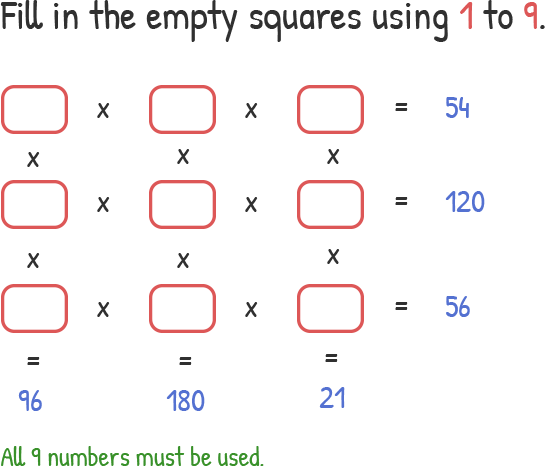We need to put numbers from 1 to 9 to complete the multiplication puzzle without repeating any numbers.

Firstly, let us look at the bottom values:

Column 1: Divisors of 96  =  1, 3, 4, 6, 8, 12, 16, 24, 96

Column 2: Divisors of 180 = 1, 2, 3, 4, 5, 6, 9, 12, 15, 18, 20, 45, 90, 180

Column 3: Divisors of 21 = 1, 3, 7, 21

We find three blocks corresponding to the following.

Row 1:  54

Row 2: 120

Row 3: 56

Here, the number we fill in each column, we get the answer below, which is 96, 180, and 21 respectively.

Step 1: Since 21 is 7 * 3 and we don’t find a multiple of 7 in rows 1 and 2, i.e., 54 and 120, we mark ‘7’ in row 3.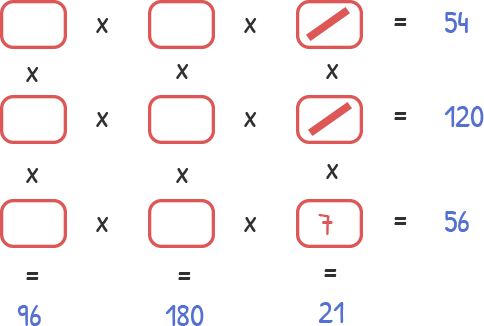However, ‘3’ is a divisor of both 54 and 120. For this, we will apply our logic in step 2.

Step 2: In Row 3, we see that 7 * 8 gives 56. Divisors of 8 are 1, 2, 4, and 8. Also, we have put such a number in the middle square of all rows such that the product is 180. Let’s say, we put ‘2’in the first square of Row 3, ‘4’in the middle, and 7 was already there, so we have: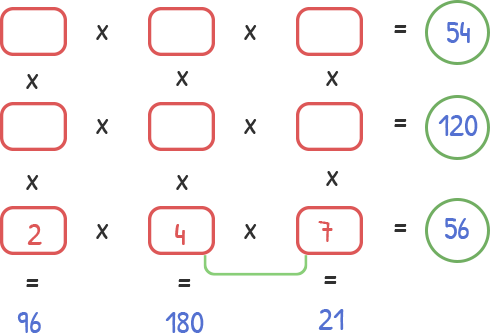So, we have 2 * 4 * 7 = 56. Now, let us look at the above two rows.

Step 3: For filling the middle column, we have to obtain 180 as our result, which is shown below: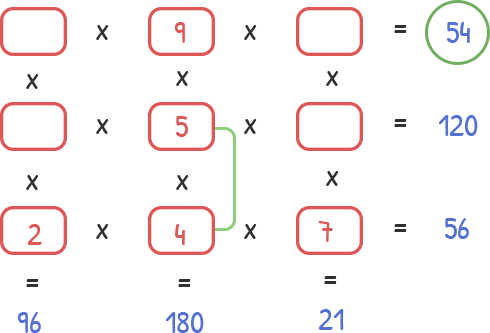Step 4: Now, in row 1, we put the following values, to obtain the row-wise result as 54.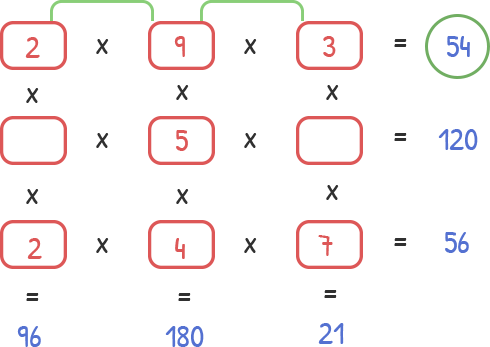Since no number must be repeated, we have to look for another arrangement to make it to 54, which is as follows.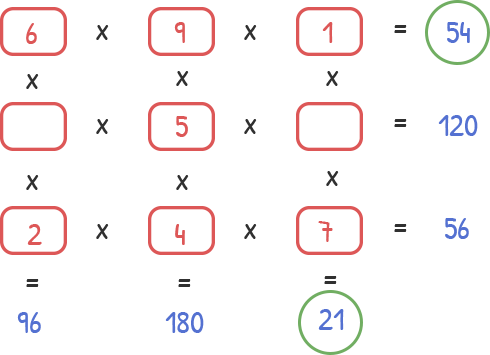Step 5: Now, we are left the middle row to get our result as 120. For this, we are left with only two numbers, i.e., 8 and 3, so let us do that as well.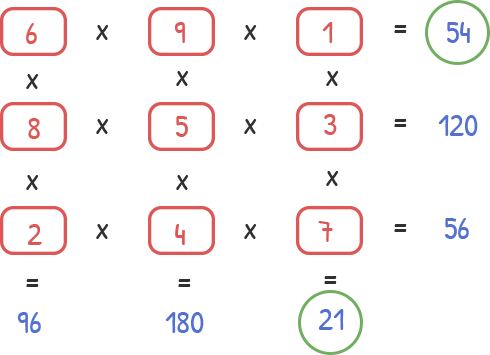Hence, our multiplication puzzle is complete.

So, this was all about the sums on multiplication for Class 4 students. Having command over this concept will help you solve a variety of questions in the simplest tricks and in lesser time.

## FAQs on Easy Tricks to Solve Multiplication Sums with Stories

1. What is multiplication?

Multiplication is a mathematical operation that we perform on a pair of numbers in order to determine a third number called the product of the two numbers. We also denote multiplication to the result of repeated additions of two numbers. An example of multiplication is 15 times 3 equals 45 or adding 15 three times give us 45.

2. Is multiply the same as repeated addition?

The multiplication is the repeated addition of two numbers that are adding as many copies of one of the two numbers, the multiplicand, as the quantity of the other one, the multiplier.

For instance, in Class 5-A, there are 5 students, in Class 5-B, there are 5 students again, then the number of students in Class 5-A. The number of students in Classes A and B will be: 5 + 5 = 10, or 5 * 2 = 10.